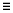# Train a Machine Learning Model

Train a machine learning model based on labeling information it contains.

Successfully training a machine learning model requires at least two labels to be defined within the selected classifier. All labels must be assigned at least three parts to train the model.
Tip: When training fails due to one or both of these reasons, use the messages displayed in the status bar to provide the missing label information and proceed with the model training.
1. From the Assembly ribbon, click the Classify > Train tool.
2. From the guide bar, select the Classifier drop-down menu to select an existing classifier to train.
3. Click Train.
After training a machine learning model, a binary file (<classifier_name>_<train_iteration>.aic) containing all training information is saved in the Classifier folder. If you add new labels to a classifier or you modify existing label assignments, retrain the machine learning model to apply the changes.
Note: The value of <train_iteration> increments by one after every successful training operation. The number of train iterations starts at 0 and the <train_iteration> suffix is appended to the file names only when train_iteration > 0.

You can use the resultant binary (.aic) files from model training on different machines. As a result, an advanced user can create the classifiers and train the machine learning models to provide files for other users to perform part classification, eliminating the need to train a classifier (See Add Trained Model File).

Tip: From the guide bar, clickand select Generate Log File, if necessary. The log files (<classifier_name>_<train_iteration>_log.txt) are saved to the Classifier folder after training a machine learning model.
After the classifier has been trained, the confusion matrix is displayed. The confusion matrix displays the accuracy of the trained machine learning model versus the labels that you provided. The confusion matrix is displayed by default. Clickto toggle the matrix on/off.

Each row shows the label name and the total number of parts given that label by the user. This sum is displayed under Support.

Each column shows the label predicted by the machine learning model.
Precision
The ratio of the number of correctly predicted labels of a given type to the total number of predictions of that same label. Provides insight into the ability of the machine learning model to predict the label correctly.
Support
The total number of parts with each label (user defined).
Overall Accuracy
The total ratio of correct predictions to the total number of predictions.
For example, refer to Figure 2.

The Floor row displays a "4" under the Floor column and "1" under the Lights column. This means there were five Floor labels given to parts by the user, as confirmed by the total in the Support column.

A "4" in the Floor column indicates that the machine learning model predicted the Floor label to have four parts. The precision is 1 because each time it predicted that a part was a Floor, it was correct.

For the Lights row, the number "7" in the Support column indicates that there were seven assigned Light labels to parts. However, the Lights column display "1" under Floor and "7" under Lights. This means the machine learning model predicted eight parts to be lights; seven times it was correct, but once it predicted a part to be a light that was assigned the label Floor. Therefore, the precision of predicting Lights is 7/8, or 0.88.

The overall accuracy value is calculated as the ratio of the sum of the confusion matrix’s diagonal entries to the column sum of the support column, therefore:(1)
$\frac{3+7+4+7+10+8+8}{3+7+5+7+10+8+8}=\frac{47}{48}\cong 0.98$
Note: Training results can be affected by the order that parts are labeled. This effect is much less pronounced as the number of parts assigned to each label increases.